# Volume 6, Number 1, Fall 2005

Detection and Analysis of Explosive's THz Spectra

Ali Setoodehnia, Feng Huang, Kamal Shahrabi

Technology Department, Kean University, Union , NJ

asetoode@kean.edu

Hong Li

New York City College of Technology, CUNY, Brooklyn, NY

Abstract

Neural network, a nonlinear mathematical approximation model, has demonstrated wide applications for classifying various lethal substances using far infrared spectral features and Terahertz Time Domain Spectroscopy (THz-TDS). It provides the optical response of a medium in both amplitude and phase. This paper presents research on an application of neural network, which leads to a detailed analysis of optical properties of explosive materials.  This process is normally complicated due to the complication of system and multiple effects involved. The research result can be applied in applications for standoff detection of the presence of explosive materials. We proposed a match filter for detection of such material in the first stage. A further investigation using neural network will be discussed.

Introduction

Due to the growing challenge of international security, many methods have been developed to monitor, detect, and characterize hidden lethal agents, such as plastic explosives strapped to a person or a bioagent in envelopes.  Terahertz radiation 1-3 spans the far infrared range with wavelengths ~ 0.3 mm. It typically transmits through plastics, paper products, etc. and may be useful for probing inside of clothing, luggage, and parcels. THz pulse spectroscopy was used to study the polymorph crystalline structures of the antihistamine drug ranitidine hydrochloride.4  Several common energetic (explosive) materials including 1,3,5 trinitro-s-triazine (RDX) have characteristic spectral features in this range.5 Reflective or transmissive THz spectra are claimed for several explosives, 5,6 bioagent simulants, and DNA. 7 The THz spectra of the explosives appear to be distinct from the spectra of human skin and material such as plastics and cloth. 5 It shows vibration features associated with intermolecular interaction relative motions 8-11 from Terahertz time domain spectroscopy (TDS), Fourier Transform Infrared (FTIR) experiments, and computer simulation of the far-infrared spectra of organic molecules.

In measurement of optical material, the standard transmission spectroscopy method cannot independently determine optical constants of the material from the transmittance of such material. In real applications, fingerprint features are indistinguishable from the barrier’s material features without the detailed knowledge of the optical constants of such material. An application of ultrafast technique allows simultaneous measurements of the thickness and optical constants of the material.  With this technique, it is possible to detect explosive material inside a common package of materials by employing a correlation detector with the available optical constants. The detector in the frequency range of far infrared can be an ideal sensor for identifying various terror attacks.

The generated and detected far infrared signal by the detector using the low temperature growth GaAs (LT-GaAs) can be expressed as: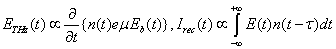(1)

Optical Properties and Correlation Detection

For a lamina material with film thickness l on top of substrate 3, the transmitted THz field with multiple reflections is given by 12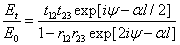.                                                           (2)

ψ=2pnl.                                                                                            (3)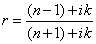.                                                                                (4)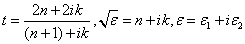,                                           (5)

where d<<c/w (for the micron film l<< 300 mm of 1 THz). Therefore, ψ <<1; n and k are refractive index and extinction coefficient, respectively, and e1 and e2 are real and imaginary parts of the dielectric constant. According to the Beer-Lambert law,

I=I0exp(-al), a=2wk/c.                                                                    (6)

To disentangle the mixed responses of two materials, apply the differential time-domain spectroscopy through small signal simplification:

Ediff=Et -Eref.                                                                                     (7)

where the Eref refers to a reference electric field incident on the substrate only. Compared to the incident wave, Eref can be expressed as

Eref=t13E0(w)exp(iwd/c).                                                                  (8)

This method works well for thin film material with very thin thickness, in the order of micrometers. The phase shift introduced by such film is assumed to be very small. For the material in the remote sensing and noninvasive detection, the condition usually is hard to meet and expect.

The following figures show the transmittance measurement using the THz-echo analysis study. As an example, consider the case of high resistive silicon, which has low absorption coefficient in the THz range, and then apply the method to other explosive materials. Consider the experimental geometry of Figure 1; the resulting THz waveform is shown to the right of Figure 1.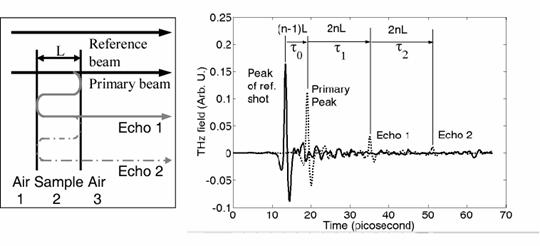Figure 1: THz and pulse echo analysis method, where the THz echo analysis method uses a silicon wafer.

The reference signal is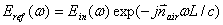(9)

where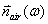(arrow implies that the value is a complex constant) the complex index of refraction of air is nearly a constant number, except the sharp features from water absorption. The primary THz pulse through the sample can be expressed as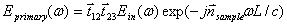(10)

The echo (first multiple reflections) through the sample is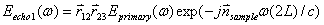(11)

where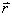and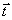are the reflection and transmission coefficients on the interface, which are determined by the Fresnel equations (normal incidence).

Dividing (2) by (1) and dividing (3) by (2), one obtains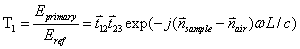(12)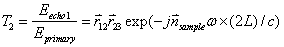(13)

(12) and (13) are unusual approaches used for determining the system transfer function of a lamella. A usual transfer function of a material should take into account the multiple reflections at the same place. However, such transfer function is not advantageous in THz approach, where the various contributions may be separable in the time domain. Phase shift from sample interfaces can be accounted for as an almost constant value compared to the contributions from the thickness dependent term in T1 and T2.

In summary from the above equations, the phase of T1 (ÐT1) is determined by the optical path length difference: (nsample-nair)L; and the ÐT2, contrary to one’s intuition, has actually different information as 2nsampleL. When phase information from the primary pulse and echo are combined, the material thickness can be extracted directly. The sample thickness is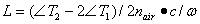(14)

After the thickness is measured directly with THz echo analysis method, the match filter can be constructed directly according to the noise n(t) and the measured T value,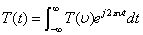(15)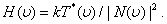(16)

If a filter transparency of amplitude transmittance T(n) multiplies the detected spectrum, the output is proportional to the amplitude transmittance of the filter.

The output can be represented as the convolution of the input with an impulse response h(t) of the match filter: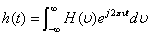(17)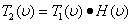(18)

The above thickness estimation is useful for a completely known response of the material. However, the multiple layers reflect the signal multiple times, and a limited amount of signal precondition is necessary. A problem might arise when applying this technique that the limitation from the thickness of the material is unknown, and therefore the matched filter is not complete without the thickness information. As a result,  the application of Neural Network in pattern recognition  is investigated in the following section.

To estimate the performance of THz imaging system, a related question is how complicated the system under study can be. One can assume a spectrum of chemical mixture with contributions from different types of materials, with concentration as a random variable set to be determined. Without losing generality, we assume normal distributions of various materials, with standard deviations from an estimated concentration. In real situations, time and temperature variation of the environment will result in a change of spectral distribution. The spectral detection is therefore time and environment dependent; a fast timing gated is generally required, and the following speckle analysis will yield the different concentration information. The detected spectra depend on the distribution of various materials. The resulting spectra are then weighted spectra of various material; a linear relation is expected since THz-TDS probes linear response. The detected response of the biological system is a linear relation: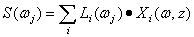(19)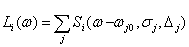(20)

where Xi(w, Z) is concentration for the ith material with standard concentration. The frequency dependence of concentration X (w, z) takes into account concentration dependent loss other than absorption; z is the depth inside the material and can be distinguished by the linear portion of time of flight information. In most cases, the frequency dependence of X can be approximated as constant value. Si is the characteristic spectrum of the ith material. Determined by the line shape used in the simulation or numerical curve fitting, Si usually included three parameters: the oscillation strength Dj, the decaying parameter sj and the center frequency wj. Three measured points are needed for uniquely determined three parameters of each oscillator. The concentration set {Xi} is the concentration solution for a particular mixture based on the known spectrum library, assuming a correlation detector is used: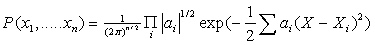(21)

The information density is determined as: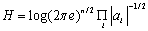(22)

For the simplest case of determining whether a component appeared or not, the success of the hypothesis is determined by the distance between the spectra mixture: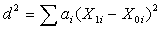(23)

where the subscript 1 means the detection of this component, and 0 means the opposite case. Assuming that the spectrometer has a spectral range Dw with a spectral resolution dw, the largest signal resulted from absorption is M, and the least signal is m, the signal will be digitized as S(wj) upon detection, and then processed by pattern recognition program. The available information capacity of such signal is: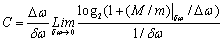(24)

### where M/m depends on the selection of Dw, the detection time for the spectra will be less than 1/dw is. Dw/dw takes into account simultaneous detection of the multiple channels signal at the same time. The system operation mode can be either a pulse method or a CW method. The capacity C of such a noisy channel should be larger than the maximum possibility of a mixture, determined by (16) and (17). From the above equations, an increase in the spectral resolution will greatly increase the channel capacity. That is a logical result when one faced an information dense subject.

A special case of detection scheme is possible with the direct detection of a particular signal in the spectral domain using the heterodyne detection, where the signal was detected only at a particular wavelength with the same specified detection period. The same channel capacity is applied in this case, except without the multiplication factor Dw/dw.

# Artificial Neural Network is a set processing element (neurons or perceptrons) with a specific topology of weighted interconnections between elements and a learning law for updating the weights of interconnection between two neurons. The complexity of ANN topology and the learning law depends on the complexity of the system. Some of the major factors that change the behavior of ANNs are number of neurons, number of interconnections and number of layers, weight schemes, threshold, and learning factors.

The roots of ANN can be traced to the work of McCulloch. In an article in 1947, Pitts and McCulloch described networks of artificial neurons capable of pattern classification. Although these networks had some computational capabilities, it was not clear how such networks could learn to perform specific tasks. The Hebbian learning rule, Delta rule and generalized Delta rule were introduced as learning algorithms later.  13,14  In 1986, Rumelhart, Hinton, and William proposed an important learning rule called the Back-Propagation Algorithm (BPA). 15 The BPA made learning possible in ANNs with multiple layers and adjustable weights.

In this paper, a supervised Feedforward Multilayer Perceptron (FMP) with BPA is used for pattern classification.  In FMP, input patterns are fed into input of multilayer and propagated forward to output layer. The output is compared with a desired output, and the error signal will be propagated backward through the network to adjust the weight of each layer using BPA. The FMP is static mapping network and requires many presentations of input patterns (data) to be learned.

The BPA is a generalized least square algorithm that minimizes the Mean Squared Error (MSE) between the desired and the actual outputs of the network with respect to weights. The basic idea is to change the weights in the direction of decreasing the total error of the ANN’s outputs.  The total error E of the network over all of the training patterns is defined as: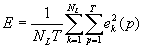(25)

where T and NL  are the total number of pattern and  neurons at output layer “L,” respectively, and ek(p) is the error associated with the pth  pattern at the output layer. The MSE used to minimize the error is expressed as: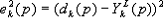(26)

where the dk(p)  and  YLk (p) are the desired and measurement outputs of the network at the kth neuron. The network weights connections Wl i,j between neurons i and j in layer l (l=1,…,L) are updated iteratively by the Generalized Delta Rule: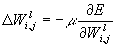(27)

where μ is the learning rate. Furthermore, using the “chain rule,” the above equation can be summarized for an output layer and hidden layer as follows:

Output Layer L: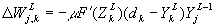(28)

where k is the neuron in the output layer and j is the neuron in the hidden layer “L-1” and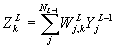(29)

Hidden layer L-1: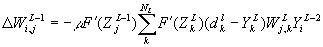(30)

The nonlinear transfer function F(Z) is sigmoid function which is differentiable and invertible.

In simulation of learning process of BPA, if the learning rate μ is small, the algorithm will approximate true parameters iteratively which converge to a global or local mini. However, if μ is too large, oscillation will result from speeding the learning process. But this can be minimal by adding a momentum term 13 to weight equation+     βΔWli,j(t-1)                                                         (31)

where β is a constant controlling the amount of momentum in the weight space.

In simulations, the data of experimental resonance frequencies for RDX given in Table 1 11 were  used as input patterns.

Table 1. Experimental resonance frequencies for RDX  11

 Experimental Peak wj (THz) aj (arb. U.) sj (THz) 1 0.79 1.21 0.11 2 1.05 0.16 0.06 3 1.34 023 0.09 4 1.44 0.11 0.11 5 1.56 0.13 0.11 6 1.77 0.06 0.12 7 1.92 0.20 0.11

where aj, wj, and sj are the oscillator strength, the frequency, and linewidth of the jth  .

With designed architecture, the Neural Network is trained with 2000 randomly generated patterns so that it identifies the patterns that closely resemble the RDX features. For this purpose, the Neural Network was trained and tested in two cases: one is without tolerance for any wandering away from the main features, and the other assumes a certain region is a more realistic testing assumption, as long as the features falling within the spherical region are defined by a 21 dimensions vector.

Experimental data have shown RDX as a material with bountiful features; it should be suitable for neural network analysis. To detect such features, one can use the mature digital identification methods. The neural network was trained to minimize the MSE to be less than 0.01. Approximately, after 200,000 or more iterations, ANN using FMP with BPA   learning method was able to identify the correct patterns for both cases. The desired outputs for accepted or rejected patterns were “1” or “0,” respectively.  Figures 2A and 2B show the results of measurement outputs and the desired output for the spherical region.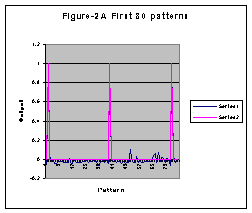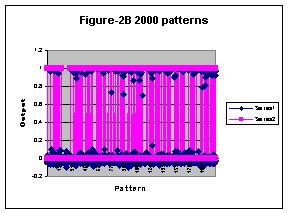Figures 2A, 2B shows the NN simulation results for 80 and 2000 patterns respectively.

Conclusion

In this research, a THz echo analysis is used as an effective way of determining the optical constants and material thickness independently. The time domain recording of THz waveform has rich spectral information that can be used to reveal depth information of an agent. A spectral domain estimation of the absorption coefficient is used in estimating the particular peak for identifying particular material.  Neural Network has been analyzed as an effective way of identifying RDX through a 21 dimension fingerprint spectral features matching. A Neural Network was designed and implemented to reduce false alarms of the detection system.

References

               D. H. Auston, K.P. Cheung, P. R. Smith, “Picosecond photoconducting Hertzian dipoles,” Appl. Phys. Lett. 45, 284 (1984)

         M. v. Exter, C. Fattinger, D. Grischkowsky, “High-brightness terahertz beams characterized with an ultrafast detector,” Appl. Phys. Lett. 55, 337 (1989)

         J. Orenstein, A. J. Millis, “Advances in the Physics of High-Temperature Superconductivity,” Science 288, 468 (2000).

         Taday, P. F.,  Bradley, I. V., Arnone, D. D., and Pepper, M., “Using Terahertz pulse spectroscopy to study the crystalline structure of a drug: A case study of the polymorphs of ranitidine hydrochloride,” Journal of Pharmaceutical Sciences, vol. 92, no. 4, pp. 831- 838 (2003).

         Kemp, M. C., P. F. Taday, B. E. Cole, J. A. Cluff, A. J. Fitzgerald, and W. R. Tribe, “Security applications of terahertz technology,” SPIE 5070 (2003).

         D. W. van derWeide (2001). “Wideband Terahertz Sensing and Spectroscopy with Electronic Sources and Detectors,” In R. E. Miles et al. (eds.), Terahertz Sources and Systems. Netherlands: Kluwer Academic Publishers (2001) 301-314.

         D. Woolard, T. Globus, E. Brown, L. Werbos, B. Gelmont, and A. Samuels, “Sensitivity Limits & Discrimination Capability of THz Transmission Spectroscopy as a Technique for Biological Agent Detection ” Proc. 5th Joint Conf. On Standoff Detection for Chemical and Biological Defense, Williamsburg, VA, Sept. 24-28 (2001).

         J. Xu, H. Liu, R. Kersting, X. Zhang, “Advancing terahertz time-domain spectroscopy for remote detection and tracing, ” Terahertz for Military and Security Applications, Proceedings of SPIE, PDS, 5070-03, Orlando, FL April 21-25 (2003).

         Heimer, T. A. and Heilweil, E. J., “Applications of Ultrafast Transient Infrared Spectroscopies,” Bulletin of the Chemical Society of Japan, vol. 75, pp. 899-908 (2002).

     F. Huang, J. Federici, D. Gary “Determining Thickness Independently from Optical Constants Using Ultrafast Light”, Optics Letter (2004) .

     F. Huang, B. Schulkin, H. Altan, J. Federici, D. Gary, R. Barat, D. Zimdars, M. Chen and D. Tanner, 'Terahertz Study of 1,3,5-Trinitro-s-triazine (RDX) by Time Domain Spectroscopy and FTIR', Appl. Phys. Lett. 85, 5535, (2004).

     F. Huang, J. Federici, and D. Gary, M. Chen, D.B.Tanner, R. Barat, D. Zimdars, “Noninvasive Study of Explosive Materials by THZ Time Domain Spectroscopy”, AIP Quantitative Nondestructive Evaluation (QNDE), (2004).

     L. Kellner, Z. phys. 56, 212 (1929); M. Born and E. Wolf, Principles of Optics, 6th Edition, Chapter 7.6 Cambridge University Press, (1980).

     Hecht-Nielson R., Neurocomputing, Addison-Wesley, 1990.

     Khanna T., Fundation of Neural Networks, Addison Wesley 1990.

     Rumelhart D., Hinton G.E., and William R.J., “Learning representation by error propagation”, In Rumelhart and McClelland, Parallel Distribution, V1 MIT, 1986.